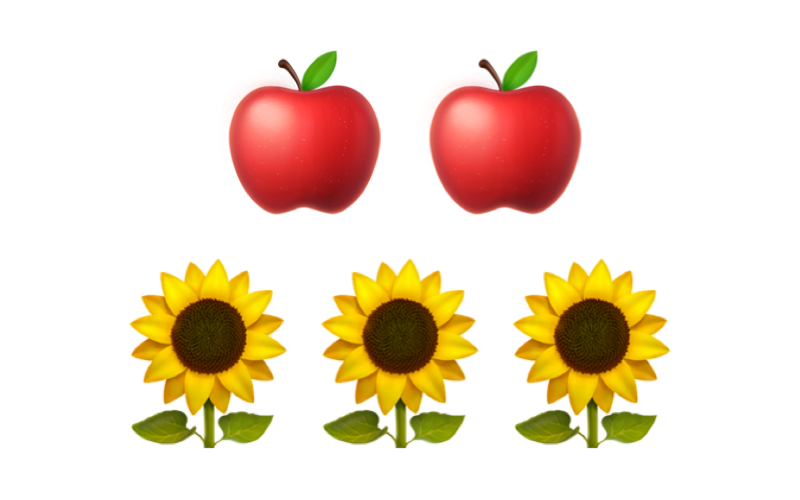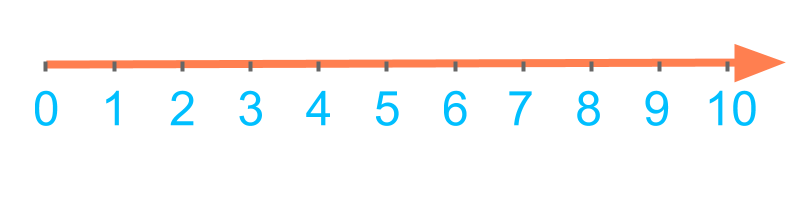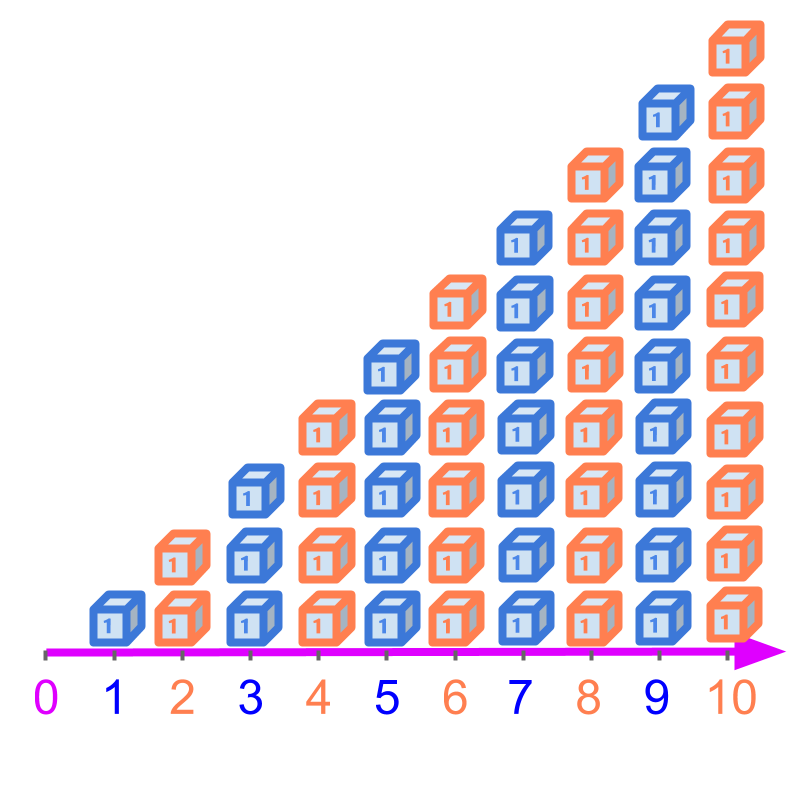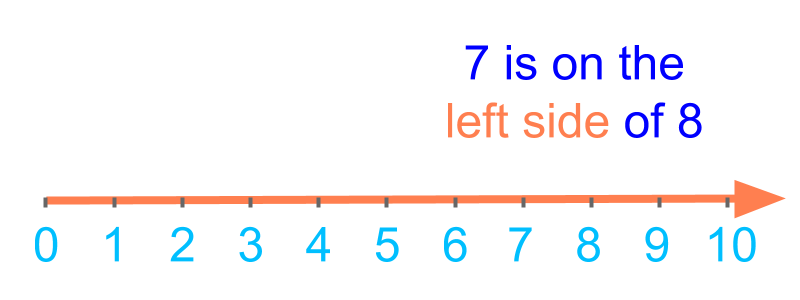maths > wholenumbers

Number-line (Whole Numbers)

what you'll learn...

overview

The ordered sequence of whole number and the representation of the order using the number-line is explained.The number of apples 2$2$ is lesser or smaller than the number of flowers 3$3$.

Such comparison is abstracted and captured as 0,1,2,$0 , 1 , 2 , \cdots$.

The following are explained. Face-value order 0<1<2<3<4<5<6<7<8<9$0 < 1 < 2 < 3 < 4 < 5 < 6 < 7 < 8 < 9$

Place-value order : units<$<$tens<$<$hundreds $< \cdots$Number-line

Ordered SequenceComparing the number of apples and number of flowers in the figure. number of flowers is more than number of apples.

Number of apples is 2, and number of flowers is 3.

The number of apples is less than the number of flowers in this given picture.

Number of apples: $2$
Number of flowers : $3$

The number of apples is less than the number of flowers in this given picture.Numbers represent count of objects or measure of quantities. The numbers are ordered with values assigned to them. The order is -- $1$, followed by $2$, followed by $3$ and so on. In the order, the value of $2$ is lesser than the value of $3$.

The word "ordered" means: to arrange in a regular way. The numbers are arranged from 0, 1, 2, et cetera.

Numbers are in an ordered sequence.
$0 , 1 , 2 , 3 , \cdots$

The ordered sequence represents the magnitude of quantity represented by the numbers. This is a fundamental property of whole numbers.

The whole numbers are in the ordered sequence

$0 , 1 , 2 , 3 , 4 , 5 , 6 , 7 , 8 , 9 , 10 , 11 ,$ etc. This is the ordinal property of whole numbers.

The word "ordinal" means: relating to order or series.The order of the numbers is represented in a line. It is called number-line. The number-line is shown in the figure.

Number order : The numbers in the whole numbers is ordered starting from $0$ to successive numbers as $0 , 1 , 2 , \cdots$

Face-value order : The order of value of a digit in a position of a number is
$0 < 1 < 2 < 3 < 4 < 5 < 6 < 7 < 8 < 9$

Place-value order : The order of place value is units$<$tens$<$hundreds `
Number Line : The order of the numbers is represented in a line.Consider comparing $8$ and $7$. The comparison of numbers in the number-line is illustrated in the figure.

The two numbers are positioned as one on the right side and another on the left side. The one on the right side is greater. The other is smaller.

summary

Number order : The numbers in the whole numbers is ordered starting from $0$ to successive numbers as $0 , 1 , 2 , \cdots$

Face-value order : The order of value of a digit in a position of a number is
$0 < 1 < 2 < 3 < 4 < 5 < 6 < 7 < 8 < 9$

Place-value order : The order of place value is units$<$tens$<$hundreds $< \cdots$Number-line : The order of the numbers is represented in a line as shown in the figure.

Outline